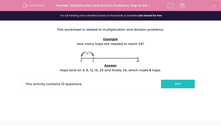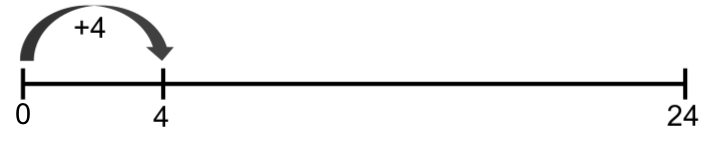# Multiplication and Division Problems: Hop to the Answer

In this worksheet, students resolve multiplication and division problems by using the idea of hopping along a number line to reach a target number.Key stage:  KS 2

Curriculum topic:   Maths and Numerical Reasoning

Curriculum subtopic:   Word Problems

Difficulty level:#### Worksheet Overview

This worksheet is related to multiplication and division problems.

Example
How many hops are needed to reach 24?